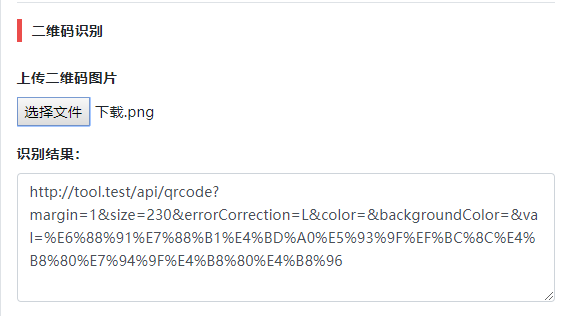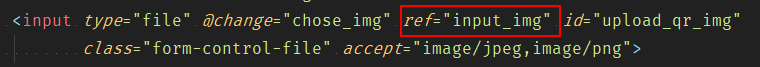# Vue中实现文件上传

### 背景### 表单### Vue中

``let form = new FormData();``

``form.append('qr_image', img.files);````````let options = {
url: 'api/qrcode/parse',
data: form,
method: 'post',
'Content-Type': 'multipart/form-data'
}
};``````

url是地址

data就是之前的FormData的实例

``````this.parse_msg = '';
axios(options).then((res) => {// do somethings});``````

### 完整代码参考

``````let img = this.\$refs.input_img;
let form = new FormData();
// console.log(img.files);
if (img.files !== undefined) {
form.append('qr_image', img.files);
let options = {
url: 'api/qrcode/parse',
data: form,
method: 'post',
'Content-Type': 'multipart/form-data'
}
};
this.parse_msg = '';
axios(options).then((res) => {
if (res.data.status === 0) {
swal({
text: res.data.msg,
icon: 'error'
})
} else {
this.parse_msg = res.data.msg;
}
});
}``````支付宝打赏微信打赏
]